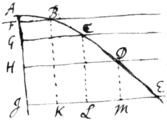<37r>

Sr

I thank you for yor kind present. Mr Andersons book is very ingenious, & may prove as usefull if his princi{illeg}|p|les be true. But I suspect one of them, namely yt ye bullet moves in a Parabola. This would be so indeed were ye horizontal celerity of ye bullet uniform, but I should think its motion decays considerably in ye flight. Suppose for instance a bullet shot horizontally moves from A moves in yeline AE, & AI being perpendicular to ye horizon in it take AF
AG, AH, AI, &c in proportion as ye square numbers 1, 4, 9, 16 &c: & its certain yt if in one moment of time ye bullet descend as low as F, in ye next moment it shall descend as low as G, in ye 3d as low as H &c. And therefore dra{illeg}|w|ing ye horizontall lines FB, GC, HD, IE; ye bullet at ye end of ye first moment will be somewhere in ye line FB suppose at B, & at ye end {illeg}|of| ye 2d moment it will be somewhere in ye line GC suppose at C &c. But that FB, GC, HD & IE are in Arithmeticall progression (wch is ye condition of ye Parabola) se{illeg}|e|ms not probable; for if it were so, ye celerity of ye bullet would increas becaus ye spaces AB, BC, CD, DE described in equall times are ye latter bigger yn ye former: whereas I should rather think yt ye celerity decreases very considerably. And perhaps this rule for its d{illeg}|ec|reasing may pretty nearly approach ye truth, viz: Letting fall ye perpendiculars BK, CL, DM &c to make IK, KL, LM &c, a decreasing Geometricall progression. If you should have occasion to speak of this to ye Author, I desire you would not mention me becaus I have no mind to concern my self further about it.

As for ye method of extracting ye roots of literal equations, the root of this ${y}^{3}+aay-{b}^{3}=0$ (wch may be a form for all cubic equations) may be thus extracted. Suppose l pretty nearly equal to ye desired root & put l in ye quotient for ye first term. Then proceed as follows.

$\begin{array}{l}\begin{array}{cc}\begin{array}{cc}\begin{array}{cc}\phantom{________________}& \phantom{__________}\end{array}& \left(l-\frac{m}{n}-\frac{3lmm}{{n}^{3}}+\frac{{m}^{3}}{{n}^{4}}\right\\ \phantom{.}\end{array}& \end{array}\\ \begin{array}{cc}\begin{array}{c}\phantom{.}\\ l+p=y& {y}^{3}& \phantom{\rule{0.2em}{0ex}}{l}^{3}+3llp+3lpp+{p}^{3}\phantom{\rule{0.5em}{0ex}}\text{.}\hfill \\ & +aay& \phantom{\rule{0.2em}{0ex}}+{a}^{2}l+aap\phantom{\rule{0.5em}{0ex}}\text{.}\hfill \\ & -{b}^{3}& -{b}^{3}\phantom{\rule{0.5em}{0ex}}\text{.}\hfill \\ \phantom{________________}& \phantom{__________}\end{array}& \begin{array}{l}\text{For brevities sake I have put}\phantom{\rule{0.5em}{0ex}}{l}^{3}+aal-{b}^{3}=m\\ \text{&}\phantom{\rule{1em}{0ex}}3ll+aa=n\phantom{\rule{0.5em}{0ex}}\text{.}\end{array}\end{array}\\ \begin{array}{cc}\begin{array}{c}\phantom{.}\\ -\frac{m}{n}+q=p& {p}^{3}& -\frac{{m}^{3}}{{n}^{3}}+\frac{3mmq}{nn}-\frac{3mqq}{n}+{q}^{3}\phantom{\rule{0.5em}{0ex}}\text{.}\hfill \\ & +3lpp& \phantom{\rule{0.2em}{0ex}}+\frac{3lmm}{nn}+\frac{6lm}{n}q+3lqq\phantom{\rule{0.5em}{0ex}}\text{.}\hfill \\ & +np& -m+nq\phantom{\rule{0.5em}{0ex}}\text{.}\hfill \\ & +m& \phantom{\rule{0.2em}{0ex}}+m\phantom{\rule{0.5em}{0ex}}\text{.}\hfill \\ \phantom{________________}& \phantom{__________}\end{array}& \end{array}\\ \begin{array}{cc}\begin{array}{c}\phantom{.}\\ -\frac{3lmm}{{n}^{3}}+r=q& {q}^{3}& -\frac{27{l}^{3}{m}^{6}}{{n}^{9}}+\frac{27ll{m}^{4}}{{n}^{6}}r-\frac{9lmm}{{n}^{3}}rr+{r}^{3}\phantom{\rule{0.5em}{0ex}}\text{.}\hfill \\ & -\frac{3m}{n}qq& -\frac{27ll{m}^{5}}{{n}^{7}}+\frac{18l{m}^{3}}{{n}^{4}}r-\frac{3m}{n}rr\hfill \\ & +3lqq& \phantom{\rule{0.2em}{0ex}}+\frac{27{l}^{3}{m}^{4}}{{n}^{6}}-\frac{18llmm}{{n}^{3}}r+3lrr\hfill \\ & +\frac{3mm}{nn}q& -\frac{9l{m}^{4}}{{n}^{5}}+\frac{3mm}{nn}r\hfill \\ & -\frac{6lm}{n}q& \phantom{\rule{0.2em}{0ex}}+\frac{18ll{m}^{3}}{{n}^{4}}-\frac{6lm}{n}r\hfill \\ & +nq& -\frac{3lmm}{nn}+nr\hfill \\ & -\frac{{m}^{3}}{{n}^{3}}& -\frac{{m}^{3}}{{n}^{3}}\phantom{\rule{0.5em}{0ex}}\text{.}\hfill \\ & +\frac{3lmm}{nn}& \phantom{\rule{0.2em}{0ex}}+\frac{3lmm}{nn}\phantom{\rule{0.5em}{0ex}}\text{.}\hfill \\ \phantom{________________}& \phantom{__________}\end{array}& \end{array}\end{array}$

<37v>

There may be other ways of extracting ye root of this equation: as for instance if b be greater then a, the root may be thus exprest $y={b}^{3}-\frac{aa}{3b}+\frac{{a}^{6}}{81{b}^{5}}$$+\frac{{a}^{8}}{243{b}^{7}}\phantom{\rule{0.5em}{0ex}}\text{&c}$. Or thus $y=\frac{{b}^{3}}{aa}-\frac{{b}^{9}}{{a}^{8}}+\frac{3{b}^{15}}{{a}^{14}}-\frac{12{b}^{21}}{{a}^{20}}\phantom{\rule{0.5em}{0ex}}\text{&c}$ if a be considerably greater then b.

The extraction of ye simple cube root may be done after ye same manner omitting only ye 2d terme $aay$. Or els it may be done as in ye following example |numerall Arithmetic|. Suppose ${y}^{3}={a}^{3}+{b}^{3}$, & that a is bigger then b, & let ye divisor for finding ye terms of ye Quotient be always tripp|l|e ye square of ye first term, that is $3aa$. And ye form of ye work will be this.

$\begin{array}{l}\begin{array}{lllll}{a}^{3}& +& {b}^{3}& \phantom{\rule{0.5em}{0ex}}& \left(a+\frac{{b}^{3}}{3aa}-\frac{{b}^{6}}{9{a}^{5}}+\frac{5{b}^{9}}{81{a}^{8}}\phantom{\rule{0.5em}{0ex}}\text{&c}\right\end{array}\\ \begin{array}{lll}{a}^{3}& & \\ 0& +& {b}^{3}\end{array}\\ \begin{array}{lll}\phantom{{0}^{0}}& \phantom{+}& \begin{array}{lllll}{b}^{3}& +& \frac{{b}^{6}}{3{a}^{3}}& +& \frac{{b}^{9}}{27{a}^{6}}\\ 0& -& \frac{{b}^{6}}{3{a}^{3}}& -& \frac{{b}^{9}}{27{a}^{6}}\end{array}\end{array}\\ \begin{array}{llll}\phantom{{0}^{0}}& \phantom{+}& \phantom{{0}^{0}}& \begin{array}{llllllllll}-& \frac{{b}^{6}}{3{a}^{3}}& -& \frac{2{b}^{9}}{9{a}^{6}}& \phantom{\rule{0.5em}{0ex}}& \ast & +& \frac{{b}^{15}}{81{a}^{12}}& +& \frac{{b}^{18}}{729{a}^{15}}\\ & \hfill 0\hfill & -& \frac{5{b}^{9}}{27{a}^{6}}& \phantom{\rule{0.5em}{0ex}}& \ast & -& \frac{{b}^{15}}{81{a}^{12}}& -& \frac{{b}^{18}}{729{a}^{15}}\end{array}\end{array}\end{array}$

So if ${y}^{3}=c$, suppose any letter as a to be pretty nearly equall to ye cube root of c, & putting $c-{a}^{3}=b$ or ${a}^{3}+b=c$ extract ye cube root out of ${a}^{3}+b$ as in ye former example; wch root $\left(\text{viz:}\phantom{\rule{0.5em}{0ex}}a+\frac{{b}^{3}}{3aa}-\frac{{b}^{6}}{9{a}^{5}}\right$$+\frac{5{b}^{9}}{81{a}^{8}}\phantom{\rule{0.5em}{0ex}}\text{&c})$ being once extracted may be kept as a rule {illeg}|f|or extracting numeral cube roots. But yet (as you suggest) these infinite series are only usefull where ye roots of equations cannot be attained accurately. For when they can be accurately attaind recours must be had to other methods, this only performing it by approximation.

Sr I am

Yor obliged humble Servant

Is. Newton

Cambridge. June 20.
1674.

<37av>

These

For Mr John Collins at the
Farthing Office in Fenchurch
Street in

London.

|2|

Parabola and extracting of
rootes in Species|